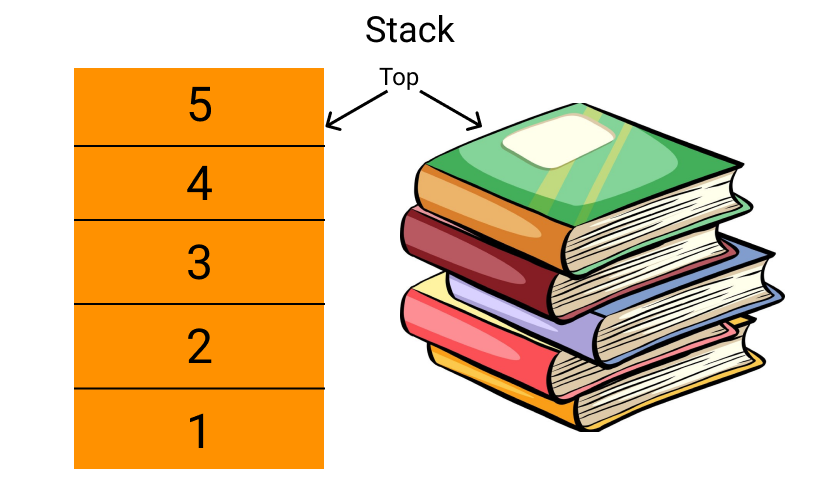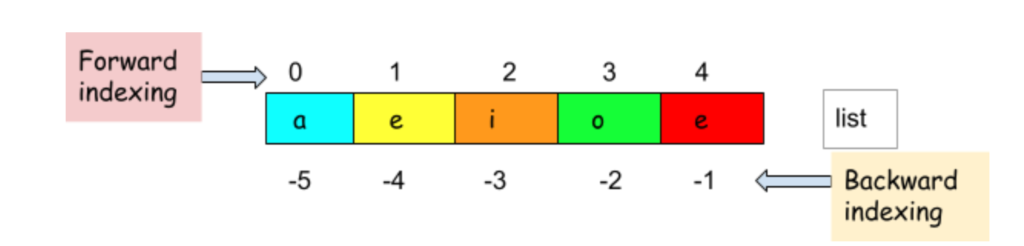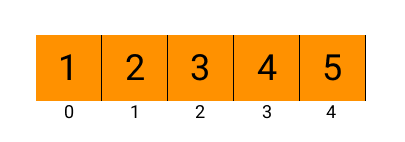# Stack in Python (LIFO Queue)Neelakshi Lahiri
Last Updated: May 13, 2022
Difficulty Level :
EASY

## Introduction

What is the first thing that comes to your mind when you hear the word stack?Source: giphy

You’re probably thinking of a stack of books, aren’t you? Well, the data structure stack is similar to a stack of books.

Like a pile of books, a data structure is used to store data in an organised manner to be accessed easily.

While picking up a book from a stack, is it possible to pull out a book from the middle without toppling the entire pile?

It isn’t. We have to go through all the books on top to get one from the middle. In other words, the last book added to the pile will be the first one picked up.

Similarly, a stack is a data structure that organises data such that the data last entered is accessed first. This is known as Last IFirst Out or LIFO.

Thus, to define it,

A stack is a data structure that works on the Last In First Out (LIFO) principle.There are various functions associated with stacks. They are:

The stack data structure and all the associated functions can be implemented in most programming languages, including the ones commonly used by us like C, C++, Java and Python. In this article, we will learn about a stack in Python.

## Stack in Python Using a List

Lists are an essential feature in Python used to store data of different types together like an array. So, lists can store a block of data and can therefore be used as a stack.

To create a stack using a list, we will simply create a list as we usually do.

We discussed some functions associated with a stack previously. So now you may be wondering how we implement those functions in a stack in Python?

Let’s find out!

## Implementation of the Standard Stack Functions

### empty( )

This method is used to check if the stack is empty.

While creating a stack in Python with lists, we will use the bool( ) method, which takes a list as a parameter. This method returns a boolean value depending on whether the list is empty.

For example,

Output:

Here the output is True since the stack contains data.

Output:

Here the output is false since the stack is empty.

### size( )

The size of a list can be found by using the len( ) method.

Similarly, the stack size in Python can be found using the same method since our stack here is a list.

Output:

### top( )

To print the last element in the stack, we will use its index as shown below:

Remember: List supports two-way indexing as shown below.Both print statements will give the same output:

Here, len(stack) gives us the length of the stack, i.e., 5. So the element present in the index 5 - 1 = 4 in the list will be printed. That element is the last, as seen below.

### push( )To add an element to a stack in Python, we will have to add it to the end of our list. To do that, there is a predefined method in Python - append( )

Output:

### pop( )

In Python, a pop( ) method takes an index as a parameter and removes the element in that index from the list.

In a stack in Python, we want to remove only the last element. So, to do that, we won’t give any parameter to the function, and the last element in the list will be popped as it happens in a stack.

Output:

Implementing a stack in Python with a list may seem easy, but it has a big disadvantage.

Like arrays, lists store the data in contiguous memory locations. So, if a lot of data is added to the stack, it may grow bigger than its memory block. Due to this reason, the working of the stack may slow down.

To overcome this problem, there is a faster alternative.

Let us see what it is next!

## Stack in Python Using the Deque Class

Python provides a container class used to store collections of data - the collection module. There is a deque class in the collection module that we can use to implement stacks in Python. The general syntax for that is:

After creating the stack, we will want to add elements to it. The method to push elements into a stack is the same as that in lists.

The rest of the standard methods in a stack using deque are the same as a list! Let’s see it for ourselves in the code below:

Output:

With this, we finish learning how to use a list and the deque class as a stack in Python, but are these the only way?Source: giphy

Let us learn the next method then!

## Stack in Python Using the Queue Module

There is another module available in Python called Queue. This can also be used to implement a stack in Python.

The queue model provides an additional feature of providing bounds to the stack.

• We can specify the size by passing a parameter maxsize to the LifoQueue( ) method. maxsize is an integer that sets the upperbound limit on the number of items that can be placed in the queue. Insertion will block once this size has been reached, until queue items are consumed. If maxsize is less than or equal to zero, the queue size is infinite.

The general syntax to create a stack using the queue module is as follows:

Let’s see how the standard methods in a stack are used here next.

## Implementation of the Standard Stack Functions

### empty( )

The empty( ) function can check if the stack is empty, as shown below. Unlike the bool( ) method we used previously, this method returns True if the stack is empty and False if it isn’t.

Output:

### size( )

Here, the qsize( ) method returns the number of elements present in the stack.

Output:

Note: Here, the stack size is 0 even though we set the max size as 5 because there are no elements in the stack. Once we push elements to the stack, the qsize( ) method returns the number of elements present.

### put( )

Here, the method to push elements to the stack is put( ). Here, if the stack has reached its maximum capacity, it will wait for space to push the element. It is used as shown below:

Output:

Another method that can be used here is put_nowait( ). This method pushes an element into the stack if there is space, and if there isn’t space, it raises an error and terminates execution.

Output:

### get( )

The get( ) method pops and returns the topmost element in the stack. This method will also wait until an element is available if it is called when the stack is empty.

Output:

get_nowait( ) method also exists, which gives an error message while popping from an empty stack.

Output:

Note: Try using the put( ) and pop( ) methods after the size of the stack has exceeded, or the stack is empty, respectively.

### full( )

An additional method exists for stacks in Python using the queue module - the full( ) function. This function checks whether the stack has reached its maximum capacity or, in other words, whether there is a maxsize number of elements in the stack.

Output:

## Complexity Analysis

The functions associated with stack are:

empty() – Returns whether the stack is empty – Time Complexity: O(1)

size() – Returns the size of the stack – Time Complexity: O(1)

top() – Returns a reference to the topmost element of the stack – Time Complexity : O(1)

push(ele) – Adds the element ‘ele’ at the top of the stack – Time Complexity : O(1)

pop() – Deletes the top most element of the stack – Time Complexity : O(1)

This brings us to the end of our Python stacks discussion. But, before we move on, did you know that Linked List can also be used to implement stacks?

Without further ado, see how to Implement Stack with Linked List and solve this frequently asked question in Amazon on CodeStudio.

Let us now see some commonly asked questions to go through what we just learned.

1. What is a stack?
A stack is a data structure that works on the Last In First Out (LIFO) principle.

2. What are the standard methods for a stack?
The standard methods for a stack are:
(i)empty( )
(ii)size( )
(iii)top( )
(iv)push( )
(v)pop( )

3. What are the different methods to implement a stack in Python?
The different methods to implement stack in Python are:
(i)Using lists
(ii)Using the Deque class
(iii)Using the Queue module

4. Is a stack in Python different from that in other programming languages?
No, a stack in Python is the same as in any other programming language.

5. How is a stack different from a queue?
A stack works on the Last In First Out (LIFO) principle, while a queue works on the First In First Out (FIFO) principle.

## Conclusion

In this article, we learned how to use a stack in Python, but what next?

Stack is used in many common competitive programming questions like:

More problems with stacks can be found here.

Other than just stacks, we can find common coding questions and the interview experience of professionals all in one place - CodeStudio.

Happy Learning!